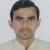true

Take Class 12 Tuition from the Best Tutors

•Affordable fees
•1-1 or Group class
•Flexible Timings
•Verified Tutors

Search in

# Circular permutationOm Prakash Mishra
24/09/2018 00

When things are arranged in a circle then number of permutations ( arrangements ) is calculated in the following way :

Since one of the objects is always fixed in its position so the total number of objects to be arranged gets reduced by 1.

So if total n objects are there then no of permutations will be

(1) when clockwise and anticlockwise permutations are counted different = (n-1)!

(2) when clockwise and anticlockwise are not counted different = (n-1)!/2

0 Dislike
Follow 3## Other Lessons for You

IDENTIFYING THE END BEHAVIOR OF A POWER FUNCTION
The coefficient is 1 (positive) and the exponent of the power function is 8 (an even number). As xx approaches infinity, the output (value of f(x)f(x) ) increases without bound. We write as x→∞,...Class 11th - Conic sections
Determine the co-ordinates (x,y) of foci for given parabola. y2 = 2(x-1)
G

How To Study Smart
Experience is the mother/father/great grandfather/mother of Education. It is a 4 step guide to build willpower and focus on taking action consistently.And taking action is the key to success...
K

IIT_Learning_Tip
Understand the concepts not formulae. Ask yourself about the problems you see due to which you cant solve a question on seeing it. So try to get rid of those problems and approach your solution in that direction.### Looking for Class 12 Tuition ?

Learn from Best Tutors on UrbanPro.

Are you a Tutor or Training Institute?

Join UrbanPro Today to find students near you
X

### Looking for Class 12 Tuition Classes?

The best tutors for Class 12 Tuition Classes are on UrbanPro

• Select the best Tutor
• Book & Attend a Free Demo
• Pay and start Learning### Take Class 12 Tuition with the Best Tutors

The best Tutors for Class 12 Tuition Classes are on UrbanPro